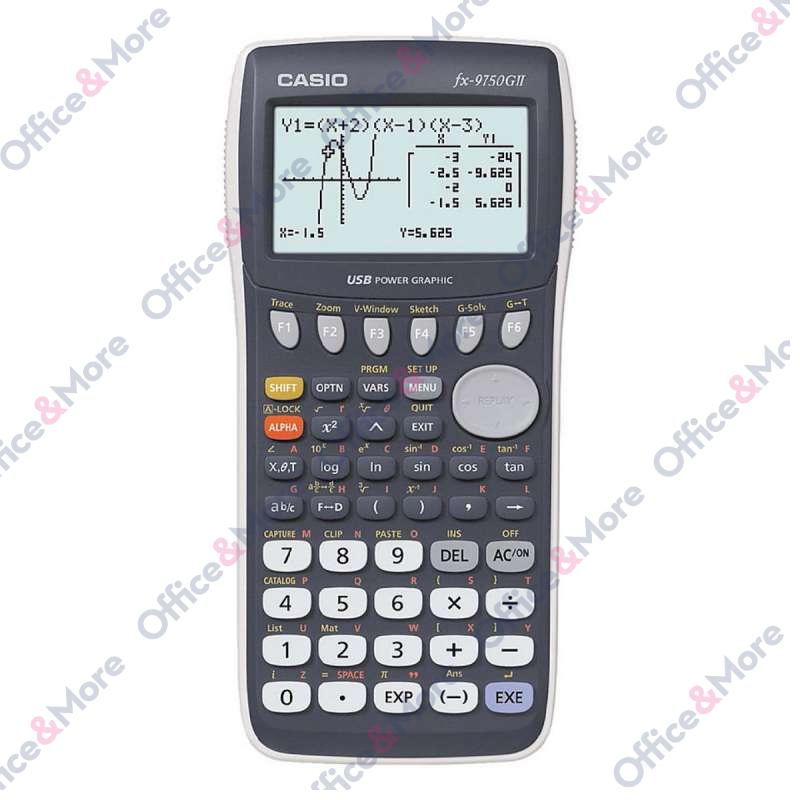CASIO

# CASIO GRAFIČKI KALKULATOR FX-9750GII

Šifra artikla: 2501150105005
Proveri dostupnost
Sačuvajte u listi želja
Features overview

61 kB program-memory
large monochrome display 8 lines by 21 characters
Size (H x W x D): 21,3 x 87,5 x 180,5 mm
Weight: 205g incl. batteries
Batteries: 4 x AAA
EQUA mode
- Solve Integral/Probability function
Ref- and Rref command lines for levels or reduced row level form
Probability function in Run Mat Application
Integral Graphing (in GRAPH mode)
Random Integer
Unit conversion
GCD (pgcd) / LCM (ppcm) function
Pie, Barchart (in STAT mode)
Mod function (Remainder)
USB
Direct connection to OHP, Casio projector
E-CON2 (Communication program for EA-200)

Functions & general information
General information:

61 kB program-memory
large monochrome display 8 lines by 21 characters
Size (H x W x D): 21,3 x 87,5 x 180,5 mm
Weight: 205g incl. batteries
Batteries: 4 x AAA

Functions:

Dynamic graphic, dual graphic
Inequations (graphic)
Parameter graphic
Polar coordinates (Graphic)
Graphic functions Trace, Plot, Line, Scroll, cutout extension, graph scale down
Calculation of value tables- and Graphic functions
Integral operations after Simpson
Calculating with complex numbers
Program-editor
Matrix operations (max. 12 x 255 resp. 255 x 12)
Calculation of linear equations (two to six variables), quadratic and cubic equations
SCI/FIX/ENG Function
26 parenthesis levels
Conversion of polar coordinates into rectangular coordinates and invers
Hyperbolic and invers hyperbolic functions
Conversion of sexagesimal into decimal and invers
Automatic fraction calculation
Random number generator
Permutation, combinatoric
Regression calculations